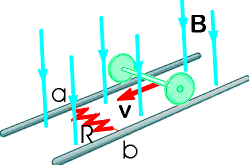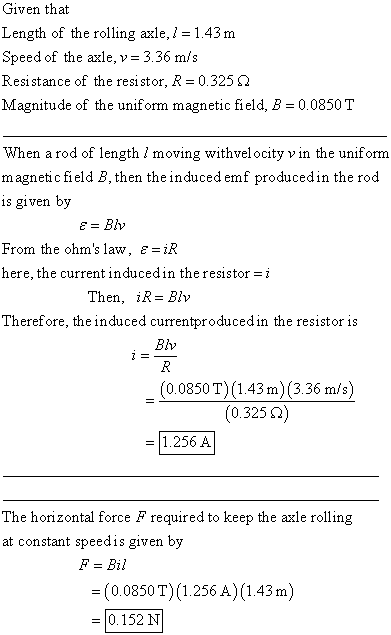# Question# In the figure below, the rolling axle, 1.43 m long, is pushed along horizontal rails at a constant speed v = 3.36 m/s.

Other
ANSWEREDIn the figure below, the rolling axle, 1.43 m long, is pushed along horizontal rails at a constant speed $$v = 3.36 m/s$$.A resistor $$R = 0.325$$ ohm is connected to the rails at points a and b, directly opposite each other. (The wheels make good electrical contact with the rails, and so the axle, rails, and R form a closed-loop circuit. The only significant resistance in the circuit is R.) There is a uniform magnetic field $$B = 0.0850 T$$vertically downward. Calculate the induced current I in the resistor and what horizontal force F is required to keep the axle rolling at constant speed?